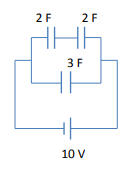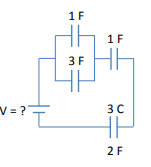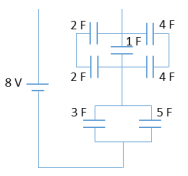Clutch Prep is now a part of Pearson
Ch 27: Capacitors & DielectricsWorksheetSee all chapters

# Solving Capacitor Circuits

See all sections
Sections
Capacitors & Capacitance
Parallel Plate Capacitors
Energy Stored by Capacitor
Capacitance Using Calculus
Combining Capacitors in Series & Parallel
Solving Capacitor Circuits
Intro To Dielectrics
How Dielectrics Work
Dielectric Breakdown

Concept #1: Solving Capacitor Circuits

Practice: What is charge and voltage across each capacitor below?Example #1: Find Charge of One Capacitor (Simple)

Practice: What is the voltage of the battery below?Practice: What is the charge on the 5 F capacitor? (hint: be careful with series vs parallel)